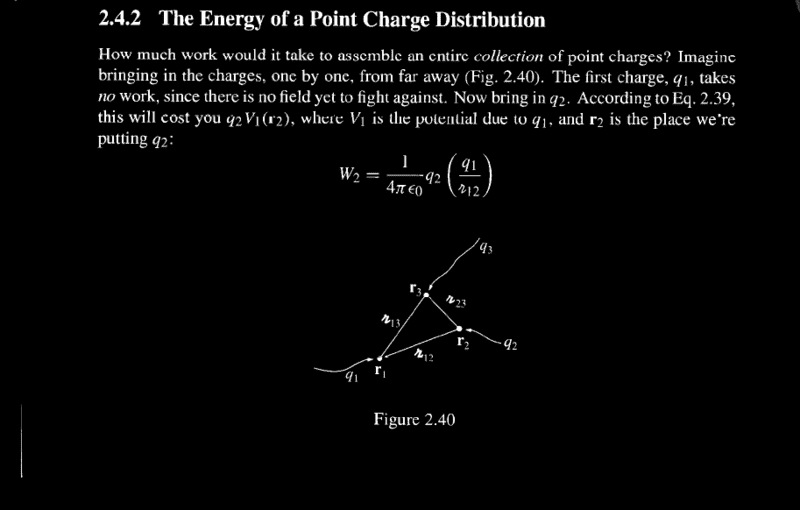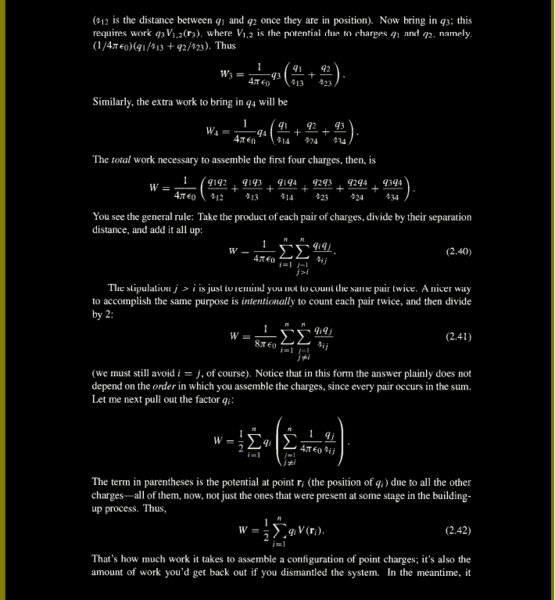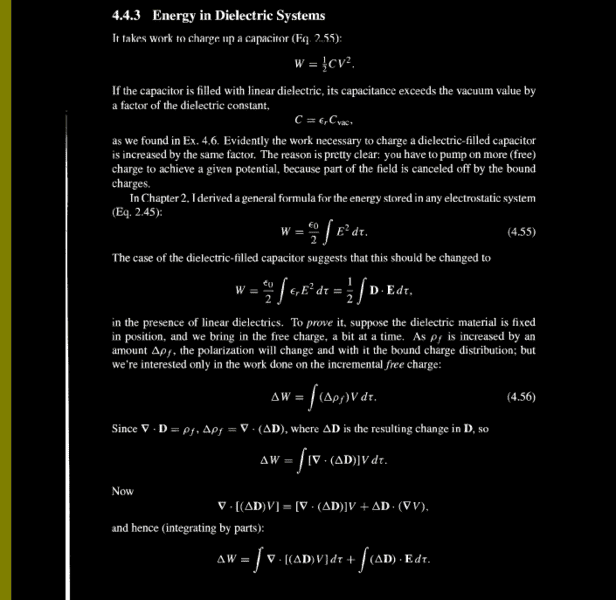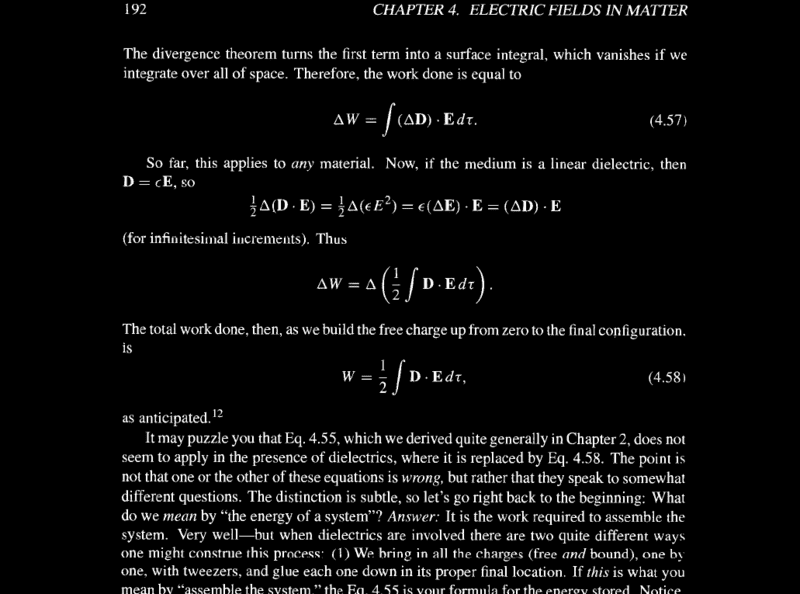# Energy stored in an electrostatic system

<Moderator's note: Thread moved from a technical forum, so homework template missing>

To do: To find an expression for energy stored in an electrostatic system with charge density ## \rho ## and volume R.
[ I am using R to denote the region filled with the given charge density as I want to keep 'V' reserved for potential ].

By the term "energy of the system " what we mean is : work required to assemble the system

So, I have to calculate work required to assemble the system i.e. W.

Let's consider the system at the time when its volume is Δ R and I bring charge dq to a point M with position vector ##\vec r ## on its surface.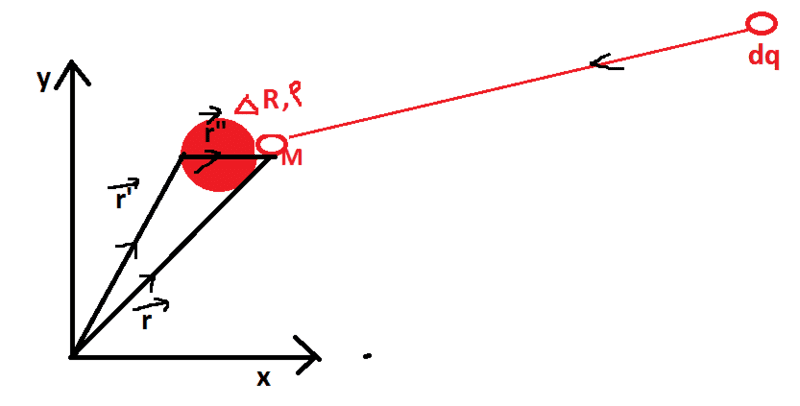Work done by me in this process is
dW = V(##\vec r ##) dq

##V \left ( \vec r \right ) = k \int _{ΔR}~ \frac { \rho (\vec {r'}) }{r"} d \, τ' ##
## dq = \rho (\vec {r}) dτ ##
So, ## dW = k \int _R~ \frac { \rho (\vec {r'}) }{r"} d \, τ' ~ \rho (\vec {r}) dτ ##
## W = \int _R~ \rho (\vec {r}) \{ k \int _{ΔR}~ \frac { \rho (\vec {r'}) }{r"} d \, τ'\} d\,τ ##

where ΔR depends on r.

Is this correct so far?

Another way:

W = ½ ##\int_R ~ \rho (\vec r) V (\vec r) d \, τ##
## \rho (\vec r) V (\vec r) = ε_0 (∇⋅ \vec E ) V = ε_0 ( ∇⋅( V \vec E ) - \vec E⋅ ∇ V ) ##
## W = ½ \int_R~ ε_0 ( ∇⋅ (V \vec E ) - \vec E⋅ ∇ V ) d \,τ ##
## = ½ \int_S ~ ε_0 V \vec E ⋅ d \vec a + ½ \int_R ε_0 E^2 d \, τ ##
Taking the region of integration to be infinite, (as the charge density outside R is 0), the first integral on R.H.S. becomes 0. Hence, we have,
## W = \frac {\epsilon _0 } 2 \int_{all space} E^2 d \, \tau ##

In case of dielectrics, this will be the work required for assembling both free charges and bound charges.

For dielectrics, ## \rho = \rho_b + \rho_f,##
So,
W = ½ ##\int_R ~ \rho (\vec r) V (\vec r) d \, τ##
= ½ ##\int_R~ (\rho_b + \rho_f )V (\vec r) d \, τ##
W =½ ## \int_R~ \rho_b V d \, τ + ½ \int_R \rho_f V (\vec r) d \, τ##

Can I take the first term on the R.H.S. as the work required to assemble the bound charges and the second term as the work required to assemble the free charges ?

If yes, then,

##W_f = ½ \int_R \rho_f V (\vec r) d \, τ
\\ \rho_f=∇.\vec D##
And doing some further calculation,
## W_f = ½ \int_{all space} \vec D . \vec E ~d \, τ ##

Is this correct so far?

#### Attachments

Last edited by a moderator:

Calculating work done required in building free charges up from zero to the final configuration in dielectrics

Here, the volume of the system( dielectrics ) R is fixed. So, as I bring free charge Δq to the system, its free charge density ## \rho_f ## gets changed by ##Δ \rho_f ##. Change in free charge density creates change in bound charge density ( only ## \rho_b ## or both## \rho_b## and ##σ_b## ?).

##Δq = \int_R (ρ_f + Δ ρ_f ) d \, τ - \int_R ρ_f d \, τ = \int_R Δρ_f d \, τ ##

Work done on bringing this incremental charge from infinity to a point with position vector ## \vec r ## inside the dielectrics is

##ΔW = Δq V(\vec r )## (1)

## ΔW = V(\vec r ) \int_R Δρ_f d \, τ ## (1.1)

But, the Griffith says in eq. 4.56 3rd ed. : ## ΔW = \int_R Δρ_f V(\vec r ) d \, τ ##
So, something may be wrong in (1).
I think Δq gets spread in all over the dielectrics. It doesn't remain at ## \vec r ## in the dielectrics.
So, eq (1) has to be modified.
Let's say that a very small amount of Δq i.e. Δ (Δq) remains at ## \vec r ## after the spreading. Now, work done on this charge is

##Δ(ΔW) = Δ(Δq) V( \vec r )##

On integrating both sides,

## ΔW = Δ\int _R Δ(Δρ_f) V(\vec r ) d \, τ ##

This also doesn't match the Griffith's expression.
I guess the way Griffith's brings the free charge is following:
He doesn't bring the charge Δq at ## \vec r ##. He brings the charge Δq over all the region of dielectrics simultaneously so that its free charge density gets increased by ## Δρ_f ##. Now, ##Δ ρ_f (\vec r ) V(\vec r ) d \, τ ## is the work done in bringing the charge ## Δ ρ_f (\vec r ) d \, τ ## at ## \vec r ##. Hence, the work done in bringing Δq all over the dielectrics is

## ΔW = \int _R~ Δ ρ_f (\vec r ) ~ V(\vec r ) d \, τ ##

Where ## V (\vec r )## is potential due to the system at ## \vec r## at the time when the charge ## Δ ρ_f (\vec r ) d \, τ ## is brought to ## \vec r##.

## Δρ_f = ∇ρ_f ⋅ d \vec r = ∇ (∇⋅ \vec D )⋅ d \vec r##

Now, Griffiths takes ## Δ ρ_f = ∇. (Δ \vec D ) ##.
This means,
## \begin {align}
Δρ_f & = Δ (∇. \vec D ) & = ∇. (Δ \vec D )
\\& ⇒ [ ∇(∇. \vec D)]. d \vec r & = ∇. ( ∇ D_j .d \vec r ) \hat x_j
\\ & ⇒ \frac { \partial { [ \frac {\partial D_j } {\partial x_j } ] } } {\partial {x_i } } d x_i & = \frac { \partial { [ \frac {\partial D_j } {\partial x_k } d x_k ] } } {\partial {x_j } }
\end {align} ##

How to prove the equality in the last equation?

Since I understand the following steps, I am copying it from Griffiths.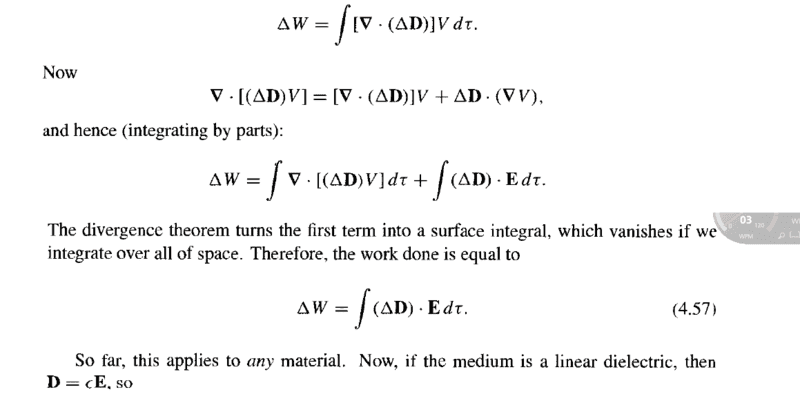Now, since we have to calculate W instead of ΔW, what Griffith does is : ## (Δ \vec D ) . \vec E = \frac {Δ(\vec D .\vec E )} 2 ##

This I can show as following:

## (Δ \vec D ) . \vec E = E_i (∇ D_i . d\vec r ) = \frac { Δ (E_i D_i )} 2 = \frac {Δ(\vec D .\vec E )} 2 ##

while taking the integration over all space, it is assumed that space outside R is vacuum.
Is this assumption necessary?

Now, Griffith takes Δ outside the integration. Can anyone please explain me how can one show the correctness of this step?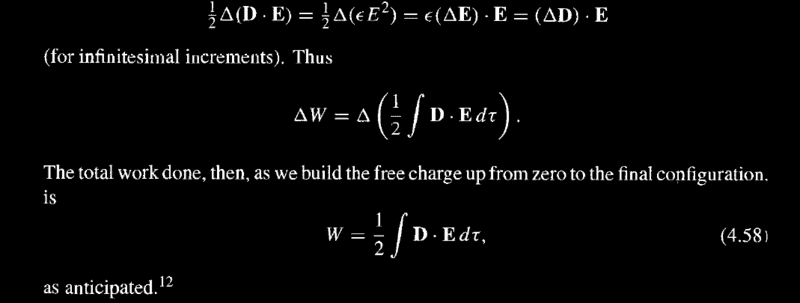Can anyone please explain me the underlined part in the following footnote?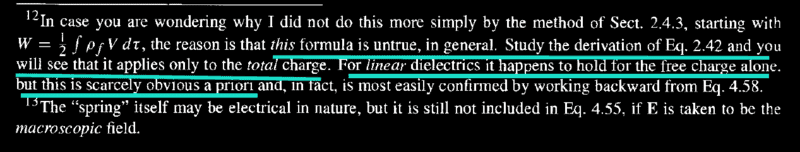I am attaching Griffith's derivation of eqn. 2.42 and 4.58 given in 3rd ed.